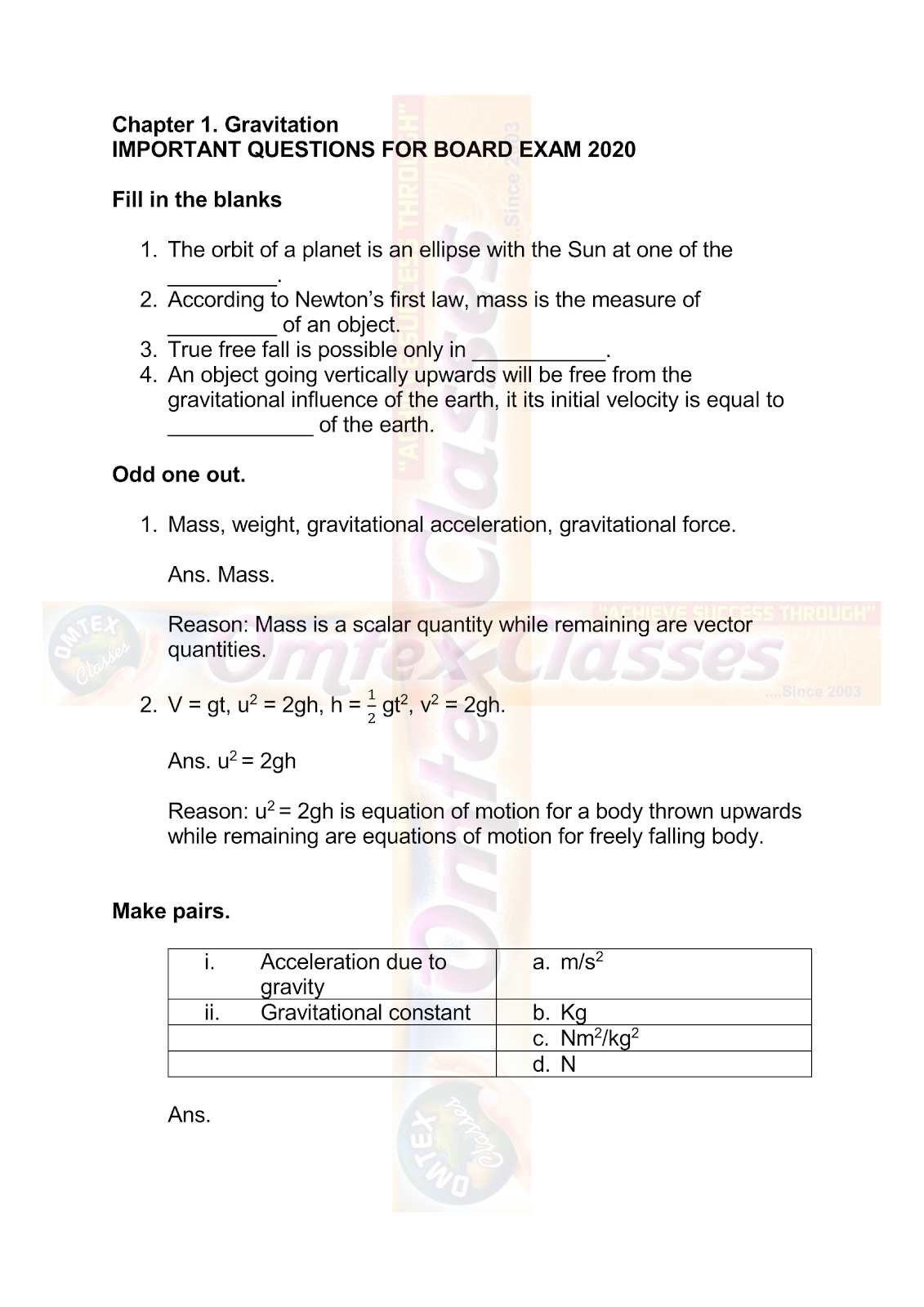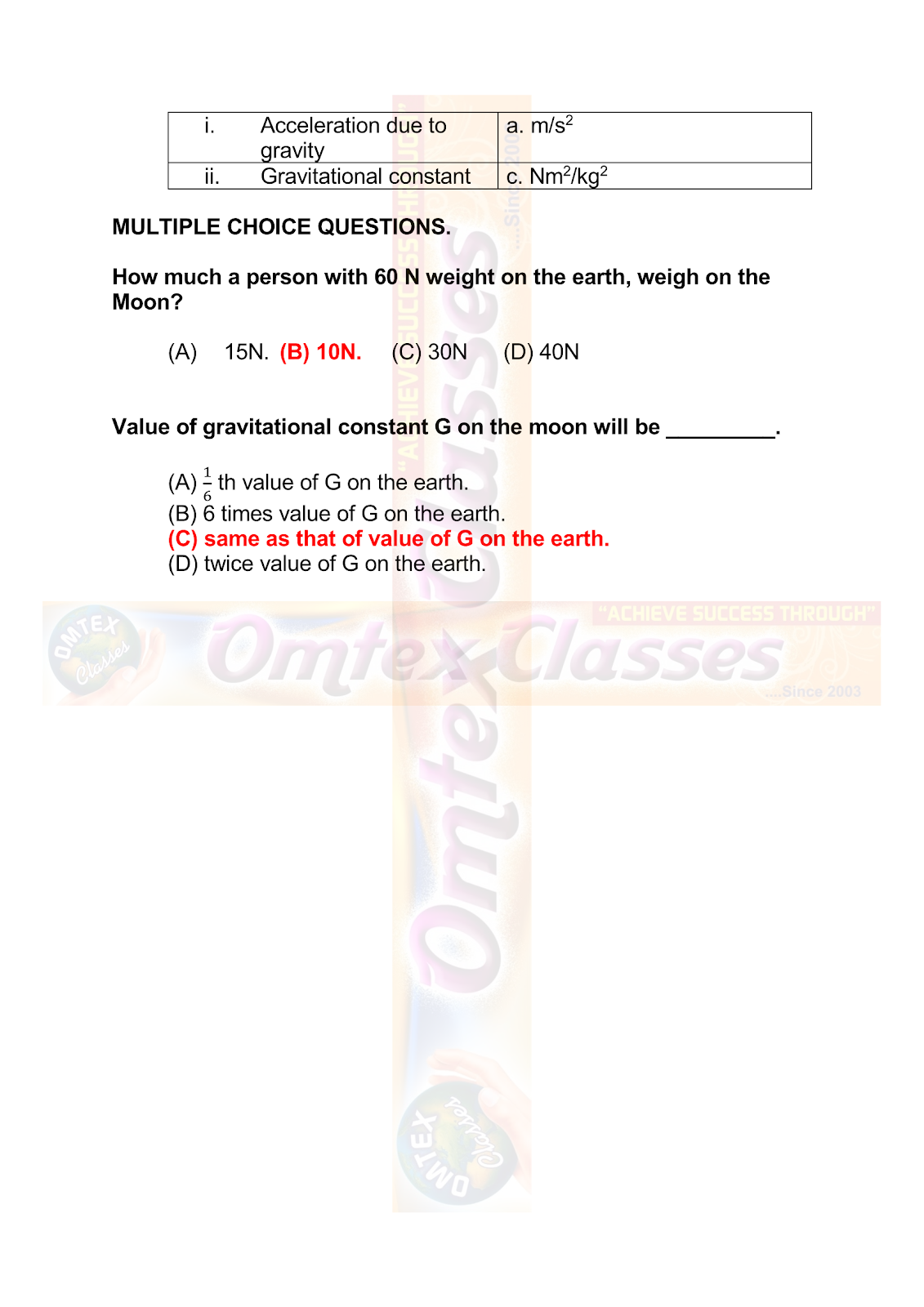SSC BOARD PAPERS IMPORTANT TOPICS COVERED FOR BOARD EXAM 2024

### GRAVITATION | SSC 10TH | MAHARASHTRA BOARD | SCIENCE PART ONE

GRAVITATION | SSC 10TH | MAHARASHTRA BOARD | SCIENCE PART ONE

5. Solve the following examples.

C. The mass and weight of an object on earth are 5 kg and 49 N respectively. What will be their values on the moon? Assume that the acceleration due to gravity on the moon is 1/6th of that on the earth. Ans. 5 kg and 8.17 N.

D. An object thrown vertically upwards reaches a height of 500 m. What was its initial velocity? How long will the object take to come back to the earth? Assume g = 10 m/s2. Ans. 100 m/s and 20 s.

E. A ball falls off a table and reaches the ground in 1 s. Assuming g = 10 m/s2, calculate its speed on reaching the ground and the height of the table. Ans. 10 m/s, and 5 m.

F. The masses of the earth and moon are 6 x 1024 kg and 7.4 x 1022 kg, respectively. The distance between them is 3.84 x 105 km. Calculate the gravitational force  of attraction between the two?
Use G = 6.7 x 10-11 N m2 kg-2 Ans. 2 x 1020 N.

G. The mass of the earth is 6 x 1024 kg. The distance between the earth and the Sun is 1.5 x 1011 m. If the gravitational force between the two is 3.5 x 1022 N, what is the mass of the Sun?
Use G = 6.7 x 10-11 N m2 kg-2   Ans. 1.96 x 1030 kg.

Projects:

Take weights of five of your friends. Find out what their weights will be on the moon and and Mars.

Gravitation Objectives